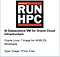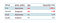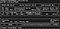# IntroductionAI Datascience VM with NVidia GPU

# Start a Notebook with TLS support.

`[opc@ml05gpu ~]\$ jupyter notebook passwordEnter password:`
`[opc@ml05gpu ~]\$ jupyter notebook — certfile=jupyter-cert.pem — keyfile=jupyter-key.key`

# Some quick initial tests.

`[opc@ml05gpu ~]\$ nvidia-smi`
`import tensorflow as tfif tf.test.gpu_device_name():    print('GPU Device: {}'.format(tf.test.gpu_device_name()))else:    print("Any troubles with GPU?")`

# Now, let’s go with H2O4GPU.

`conda create -n h2o4gpu -c h2oai -c conda-forge h2o4gpu-cuda10`
`conda activate h2o4gpu`
`conda install tornado==4.5.3conda upgrade jupyter_client`
`jupyter notebook — certfile=jupyter-cert.pem — keyfile=jupyter-key.key`
`import h2o4gpuimport numpy as npX = np.array([[1.,1.], [1.,4.], [1.,0.]])model = h2o4gpu.KMeans(n_clusters=2,random_state=1234).fit(X)model.cluster_centers_`

# Test the performance using XGBoost.

`https://github.com/h2oai/h2o4gpu/blob/master/examples/py/xgboost_simple_demo.ipynb`
`import xgboost as xgbimport numpy as npfrom sklearn.datasets import fetch_covtypefrom sklearn.model_selection import train_test_splitimport time%%time # Fetch dataset using sklearn cov = fetch_covtype() X = cov.data y = cov.target%%time# Create 0.75/0.25 train/test splitX_train, X_test, y_train, y_test = train_test_split(X, y, test_size=0.25, train_size=0.75, random_state=42)%%time# Convert input data from numpy to XGBoost formatdtrain = xgb.DMatrix(X_train, label=y_train)dtest = xgb.DMatrix(X_test, label=y_test)num_round = 10maxdepth = 6# base parametersparam = {'tree_method': 'gpu_hist',         'grow_policy': 'depthwise',         'max_depth': maxdepth,         'random_state': 1234,         'objective': 'multi:softmax', # Specify multiclass classification         'num_class': 8, # Number of possible output classes         'base_score': 0.5,         'booster': 'gbtree',         'colsample_bylevel': 1,         'colsample_bytree': 1,         'gamma': 0,         'learning_rate': 0.1,          'max_delta_step': 0,         'min_child_weight': 1,         'missing': None,         'n_estimators': 3,         'scale_pos_weight': 1,         'silent': True,         'subsample': 1,         'verbose': True,         'n_jobs': -1         }%%time# First setup: GPU HIST DEPTHWISEparam['tree_method'] = 'gpu_hist'param['grow_policy'] = 'depthwise'param['max_depth'] = maxdepthparam['max_leaves'] = 0gpu_res = {} # Store accuracy resulttmp = time.time()# Train modelxgb.train(param, dtrain, num_round, evals=[(dtest, 'test')], evals_result=gpu_res)print("GPU Training Time: %s seconds" % (str(time.time() - tmp)))%%time# Second setup: GPU HIST LOSSGUIDEparam['tree_method'] = 'gpu_hist'param['grow_policy'] = 'lossguide'param['max_depth'] = 0param['max_leaves'] = np.power(2,maxdepth)gpu_res = {} # Store accuracy resulttmp = time.time()# Train modelxgb.train(param, dtrain, num_round, evals=[(dtest, 'test')], evals_result=gpu_res)print("GPU Training Time: %s seconds" % (str(time.time() - tmp)))%%time# Third setup: CPU HIST DEPTHWISEparam['tree_method'] = 'hist'param['grow_policy'] = 'depthwise'param['max_depth'] = maxdepthparam['max_leaves'] = 0cpu_res = {} # Store accuracy resulttmp = time.time()# Train modelxgb.train(param, dtrain, num_round, evals=[(dtest, 'test')], evals_result=cpu_res)print("CPU Training Time: %s seconds" % (str(time.time() - tmp)))%time# Fourth setup: CPU HIST LOSSGUIDEparam['tree_method'] = 'hist'param['grow_policy'] = 'lossguide'param['max_depth'] = 0param['max_leaves'] = np.power(2,maxdepth)cpu_res = {} # Store accuracy resulttmp = time.time()# Train modelxgb.train(param, dtrain, num_round, evals=[(dtest, 'test')], evals_result=cpu_res)print("CPU Training Time: %s seconds" % (str(time.time() - tmp)))`Comparison of execution times, with and without GPUGPU utilization in the test

# Conclusion.

Born in the wonderful city of Naples, but living in Rome. Always curious about new technologies and new things. I work in a big Cloud Company.

## More from Luigi Saetta

Born in the wonderful city of Naples, but living in Rome. Always curious about new technologies and new things. I work in a big Cloud Company.